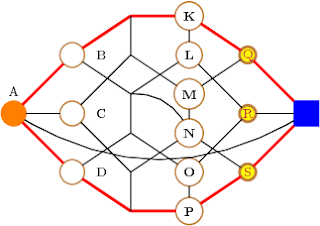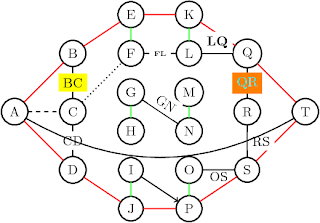## Saturday, September 05, 2009

### A 3d graph... after the example in the tikz gallery.

\usetikzlibrary{calc,3d}
\newcommand{\setxyz}{%
\pgfmathsetmacro{\xone}{cos(180+#1)}%
\pgfmathsetmacro{\yone}{sin(180+#1)}%
\pgfmathsetmacro{\xtwo}{cos(360-#1)}%
\pgfmathsetmacro{\ytwo}{sin(360-#1)}%
}
\setxyz{17}
\begin{tikzpicture}%
[x = {(\xone cm,\yone cm)},
y = {(\xtwo cm,\ytwo cm)},
z = {(0cm,1cm)}]
\SetVertexNoLabel
\begin{scope}[canvas is xy plane at z=-5]
\Vertex{x}
\end{scope}
\begin{scope}[canvas is xy plane at z=0]
\grEmptyCycle[prefix=a]{5}
\end{scope}
\EdgeFromOneToAll{x}{a}{}{5}
\Edges(a0,a1,a2,a3,a4,a0)
\begin{scope}[canvas is xy plane at z=5]
\Vertex{y}
\end{scope}
\EdgeFromOneToAll{y}{a}{}{5}
\end{tikzpicture}

## Friday, August 28, 2009

### A circulant tournamentThis is the circulant C_9(1,2,3,4)

\begin{tikzpicture}
\usepgflibrary{arrows}
\GraphInit[vstyle=Art]
\SetUpEdge[style={->,>=angle 45,bend right=10},color=red]
\grCirculant[RA=3]{9}{1,-2,3,-4}
\end{tikzpicture}

## Tuesday, August 18, 2009

### Several options for the \Vertex macro

UPDATE: This example no longer works with the latest versions of tkz-graph and tkz-berge. For an updated version see this post at my new blog.\begin{tikzpicture}
\SetVertexNormal[LineColor=brown]
\Vertex[x=0,y=2.5,style=orange,LabelOut=true,Lpos=90]{A}
{\tikzstyle{every node} = [node distance=1.5cm]
\Vertices[x=1.5,y=4,dir=\SO,LabelOut=true,Ldist=5pt]{B,C,D}}
\Vertices[x=3,y=5,dir=\SO,style={shape=coordinate}]{E,F,G,H,I,J}
\Vertices[x=4.5,y=5,dir=\SO,style={font=\bfseries}]{K,L,M,N,O,P}
{\tikzstyle{every node} = [node distance=1.5cm]
\Vertices[x=6,y=4,dir=\SO,
style={line width=2pt,
inner sep=0pt,
text=purple,
fill=yellow,
minimum size=12pt}]{Q,R,S}}
\Vertex[x=7.5,y=2.5,style={shape=rectangle,blue}]{T}
\Edges[color=red,lw=2pt](A,B,E,K,Q,T,S,P,J,D,A)
\Edges(B,G,L,R,T)
\Edges(D,H,O,R)
\Edges(A,C,F,M,Q)
\Edges(C,I,N,S)
\foreach \x/\y in {E/F,G/H,I/J,K/L,M/N,O/P}
{\Edge(\x)(\y)}
\Edge[style={bend left}](G)(N)
\Edge[style={bend right}](A)(T)
\end{tikzpicture}

## Monday, August 03, 2009

### Several options for the \Edge macro\begin{tikzpicture}
\SetVertexNormal
\Vertex[x=0,y=2.5]{A}
{\tikzstyle{every node} = [node distance=1.5cm]
\Vertices[x=1.5,y=4,dir=\SO]{B,C,D}}
\Vertices[x=3,y=5,dir=\SO]{E,F,G,H,I,J}
\Vertices[x=4.5,y=5,dir=\SO]{K,L,M,N,O,P}
{\tikzstyle{every node} = [node distance=1.5cm]
\Vertices[x=6,y=4,dir=\SO]{Q,R,S}}
\Vertex[x=7.5,y=2.5]{T}
\Edges[color=red](A,B,E,K,Q,T,S,P,J,D,A)
\foreach \x/\y in {E/F,G/H,I/J,K/L,M/N,O/P}
{\Edge[color=green](\x)(\y)}
\Edge[style=->](I)(P)
\Edge[style=dashed](A)(C)
\Edge[style=dotted](C)(F)
\Edge[label=CD](C)(D)
\Edge[label=BC,labelcolor=yellow](B)(C)
\Edge[label=QR,labelcolor=orange,labeltext=cyan](Q)(R)
\Edge[label=RS,labelstyle=right](R)(S)
\Edge[label=OS,labelstyle={below=1pt,inner sep=0pt}](O)(S)
\Edge[label=LQ,labelstyle={above,font=\bfseries}](L)(Q)
\Edge[label=FL,labelstyle={font=\tiny\bfseries}](F)(L)
\Edge[label=GN,labelstyle={sloped,above}](G)(N)
\Edge[style={bend right}](A)(T)
\end{tikzpicture}

### Simulating KTikZ with Emacs

I stumbled upon a piece of software called KtikZ. The screenshot in that page, together with the fact that Doc-View mode is included in the new Emacs 23, inspired me to try that idea with tikz2pdf. With help from folks from gnu.emacs.help, I came up with the following function:

(defun split-for-tikz2pdf ()
(interactive)
(TeX-run-style-hooks "tikz")
(TeX-run-style-hooks "tkz-berge")
(split-window-horizontally 60)
(other-window 1)
(split-window-vertically 15)
(find-file "tikz2pdf_temp.pdf")
(doc-view-mode)
(auto-revert-mode 1)
(other-window 1)
(let ((file-path (buffer-file-name)))
(when file-path
(shell)
(comint-send-string (get-buffer-process (current-buffer))
(format "tikz2pdf -v %S \n" file-path))))
)

which gives something like this, when applied at a buffer with a .tex file:Unfortunately, the buffer for the .pdf file is not in Doc-View mode after executing the function. One has to go to that window and do M-x doc-view-mode. After that, sometimes the picture is not generated correctly and one has to reload manually with g. Other useful Doc-View key bindings are + and -.

You might want to use my simple AucTeX style files tikz.el and tkz-berge.el.